# Tangent space at a point

This article defines a basic construct that makes sense on any differential manifold
View a complete list of basic constructs on differential manifolds

## Definition

Let$M$ be a differential manifold and$p \in M$. The tangent space at$p$ to$M$, denoted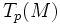$T_p(M)$, is defined in a number of equivalent ways.

### In the language of derivations

Consider the algebra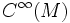$C^\infty(M)$ of infinitely differentiable functions on$M$. Then, the tangent space$T_p(M)$ is defined as the vector space of all derivations: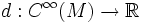$d: C^\infty(M) \to \R$

By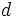$d$ being a derivation, we mean:

•$d$ is a$\R$-linear map
• Given functions$f,g \in C^\infty(M)$ we have: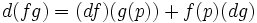$d(fg) = (df)(g(p)) + f(p)(dg)$

### In the language of curves

The tangent space$T_p(M)$ is defined, as a set, as the quotient of the set of all curves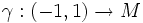$\gamma: (-1,1) \to M$ where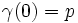$\gamma(0) = p$, by the following equivalence relation:$\gamma_1 = \gamma_2$ if, under the mapping to an open subset in$\R^n$ via a coordinate chart,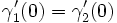$\gamma_1'(0) = \gamma_2'(0)$.

With this definition, addition is not very clear.

### In the language of local coordinate charts

In this language, the tangent space is defined as the tangent space to its image in$\R^n$ under a local coordinate chart. Fill this in later